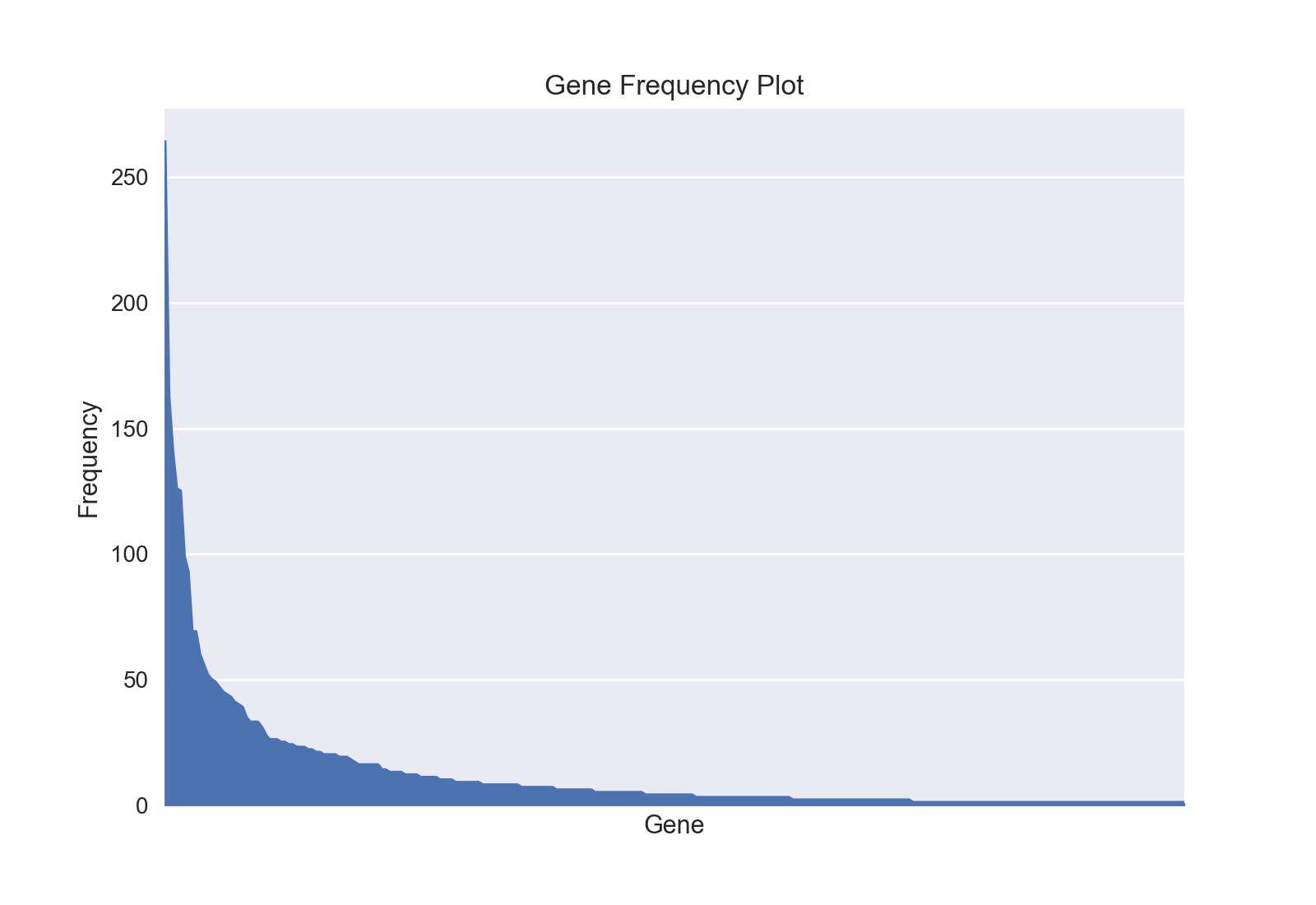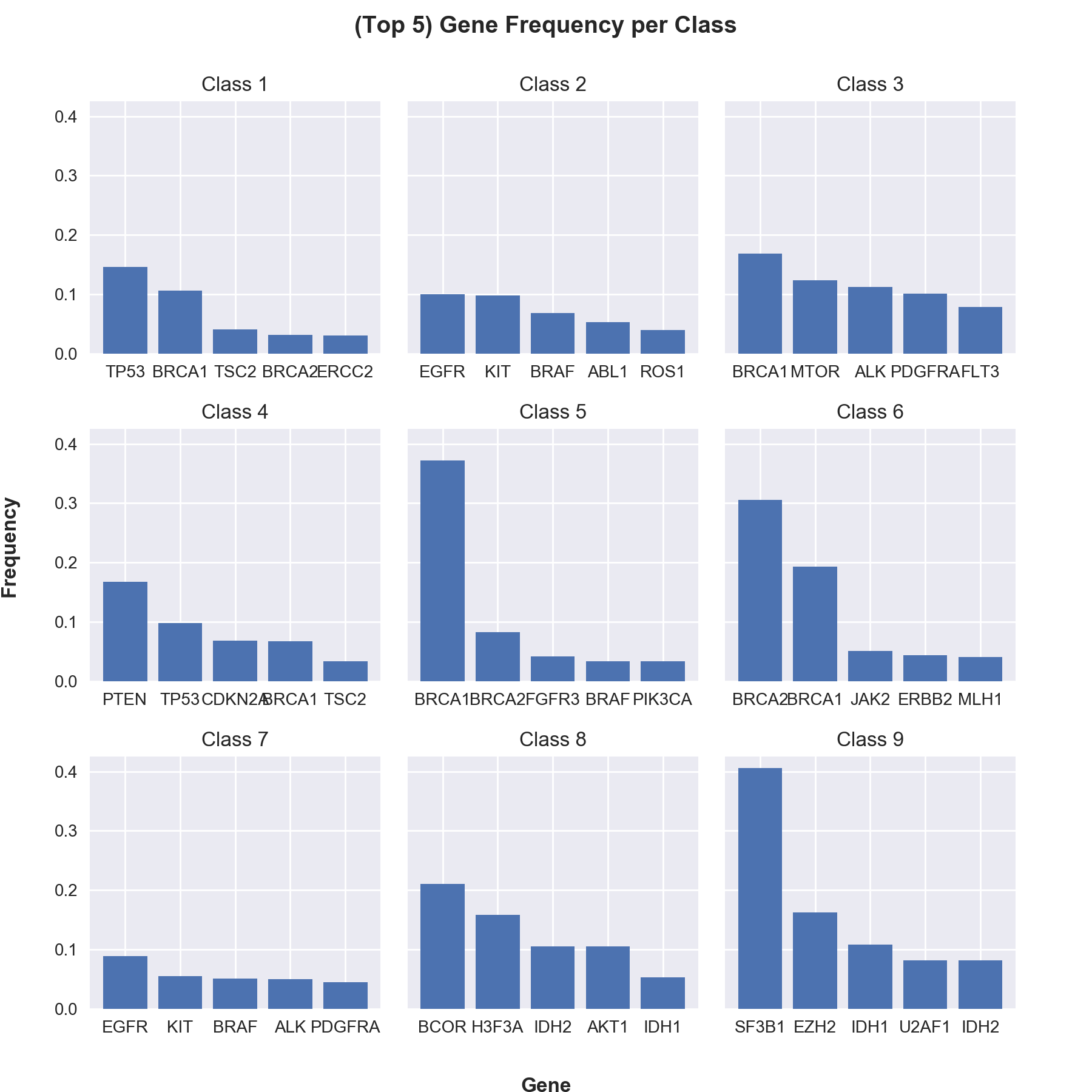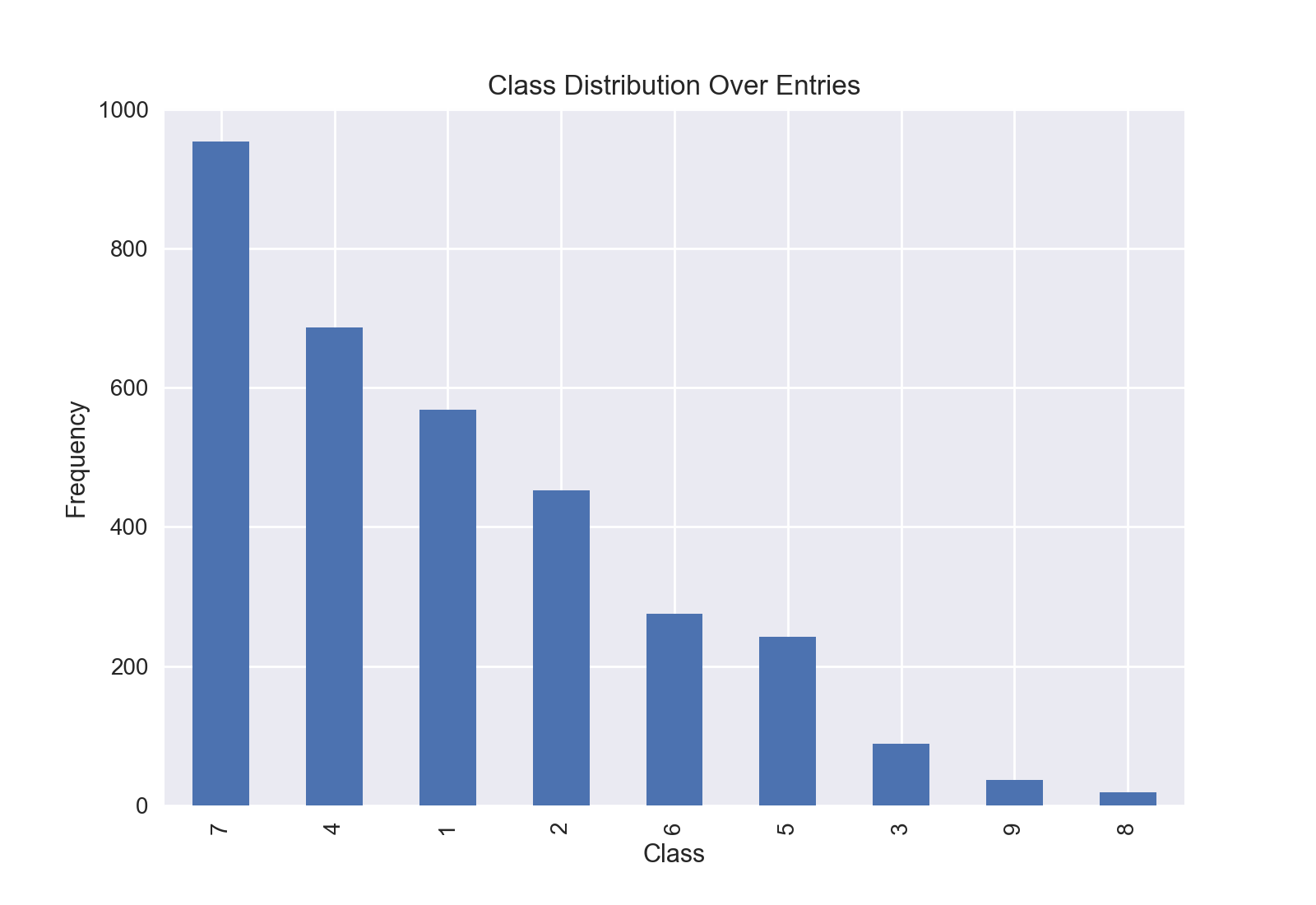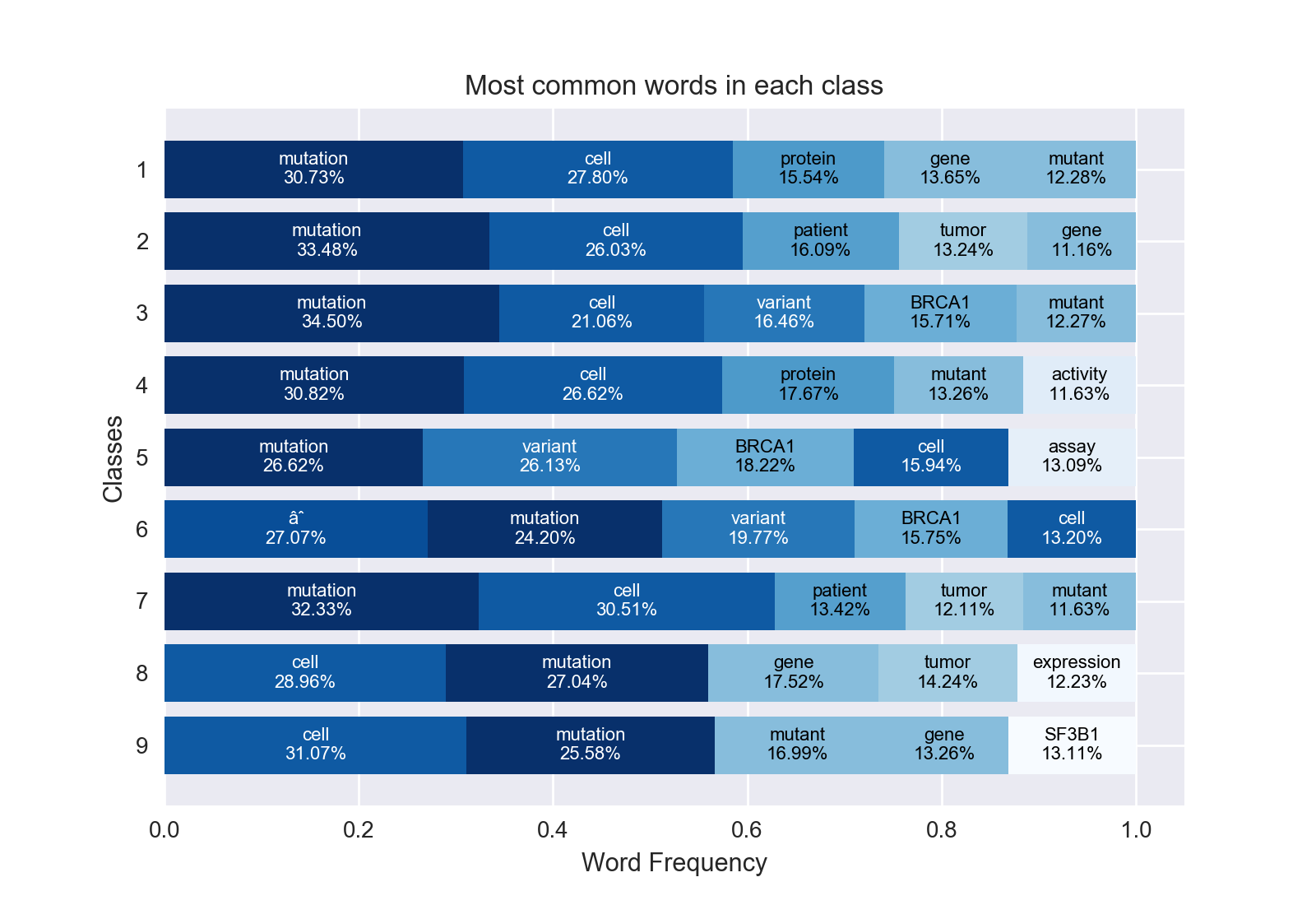# Kaggle Personalized Medicine EDA

01 Nov 2017

This repository contains a simple exploratory analysis for Personalized Medicine: Redefining Cancer Treatment competition in Kaggle. This post is a markdown version of my kernel: preliminary data analysis using word2vec from kaggle. Jupter nbconvert has been used for markdown conversion.

# A simple anaysis of the dataset using nltk and Word2Vec

This notebook goes over the dataset in the following order:

• Read the data into a dataframe using pandas library.
• Cleaning unnecessary data (unique or null columns).
• Analyzing data distributions.
• Analyzing text data via keywords and summarization.
• Tokenizing and creating a word cloud of the whole text.
• Using Word2Vec to check the correlation between text and the classes.

This kernel has been tested with python 3.6 (x64) on Windows.

``````%matplotlib notebook

# Data wrapper libraries
import pandas as pd
import numpy as np

# Visualization Libraries
import matplotlib.pyplot as plt
from matplotlib.patches import Patch
from matplotlib.markers import MarkerStyle
import seaborn as sns

# Text analysis helper libraries
from gensim.summarization import summarize
from gensim.summarization import keywords

# Text analysis helper libraries for word frequency etc..
from nltk.tokenize import word_tokenize
from nltk.stem import WordNetLemmatizer
from nltk.corpus import stopwords
from string import punctuation

# Word cloud visualization libraries
from scipy.misc import imresize
from PIL import Image
from wordcloud import WordCloud, ImageColorGenerator
from collections import Counter

# Word2Vec related libraries
from gensim.models import KeyedVectors

# Dimensionaly reduction libraries
from sklearn.decomposition import PCA

# Clustering library
from sklearn.cluster import KMeans
``````

Let’s take a casual look at the variants data.

``````df_variants = pd.read_csv('data/training_variants').set_index('ID')
``````
Gene Variation Class
ID
0 FAM58A Truncating Mutations 1
1 CBL W802* 2
2 CBL Q249E 2
3 CBL N454D 3
4 CBL L399V 4

Let’s take a look at the text data. Data is still small enough for memory so read to memory using pandas.

``````df_text = pd.read_csv('data/training_text', sep='\|\|', engine='python',
skiprows=1, names=['ID', 'Text']).set_index('ID')
``````
Text
ID
0 Cyclin-dependent kinases (CDKs) regulate a var...
1 Abstract Background Non-small cell lung canc...
2 Abstract Background Non-small cell lung canc...
3 Recent evidence has demonstrated that acquired...
4 Oncogenic mutations in the monomeric Casitas B...

Join two dataframes on index

``````df = pd.concat([df_variants, df_text], axis=1)
``````
Gene Variation Class Text
ID
0 FAM58A Truncating Mutations 1 Cyclin-dependent kinases (CDKs) regulate a var...
1 CBL W802* 2 Abstract Background Non-small cell lung canc...
2 CBL Q249E 2 Abstract Background Non-small cell lung canc...
3 CBL N454D 3 Recent evidence has demonstrated that acquired...
4 CBL L399V 4 Oncogenic mutations in the monomeric Casitas B...

Variation column is mostly consists of independant unique values. So its safe to drop.

``````df['Variation'].describe()
``````
``````count                     3321
unique                    2996
top       Truncating Mutations
freq                        93
Name: Variation, dtype: object
``````

Gene column is a bit more complicated, values seems to be heavly skewed. Data can still be valuable if normalized and balanced by weights.

``````plt.figure()
ax = df['Gene'].value_counts().plot(kind='area')

ax.get_xaxis().set_ticks([])
ax.set_title('Gene Frequency Plot')
ax.set_xlabel('Gene')
ax.set_ylabel('Frequency')

plt.show()
``````Even with domination of some gene’s, it still gives a nice insight from their class distributions.

But not to over complicate things for this kernel, we’ll skip that and drop it as well.

``````fig, axes = plt.subplots(nrows=3, ncols=3, sharey=True, figsize=(9,9))

# Normalize value counts for better comparison
def normalize_group(x):
label, repetition = x.index, x
t = sum(repetition)
r = [n/t for n in repetition]
return label, r

for idx, g in enumerate(df.groupby('Class')):
label, val = normalize_group(g["Gene"].value_counts())
ax = axes.flat[idx]
ax.bar(np.arange(5), val[:5],
tick_label=label[:5])
ax.set_title("Class {}".format(g))

fig.text(0.5, 0.97, '(Top 5) Gene Frequency per Class', ha='center', fontsize=14, fontweight='bold')
fig.text(0.5, 0, 'Gene', ha='center', fontweight='bold')
fig.text(0, 0.5, 'Frequency', va='center', rotation='vertical', fontweight='bold')
fig.tight_layout(rect=[0.03, 0.03, 0.95, 0.95])
``````And finally lets look at the class distribution.

``````plt.figure()
ax = df['Class'].value_counts().plot(kind='bar')

ax.set_title('Class Distribution Over Entries')
ax.set_xlabel('Class')
ax.set_ylabel('Frequency')

plt.show()
``````Distribution looks skewed towards some classes, there are not enough examples for classes 8 and 9. During training, this can be solved using bias weights, careful sampling in batches or simply removing some of the dominant data to equalize the field.

Finally, lets drop the columns we don’t need and be done with the initial cleaning.

``````df.drop(['Gene', 'Variation'], axis=1, inplace=True)

# Additionaly we will drop the null labeled texts too
df = df[df['Text'] != 'null']
``````

Now let’s look at the remaining data in more detail. Text is too long and detailed, so I’ve decided to summarize it using gensim. Still didn’t understand anything :/

``````t_id = 0
text = df.loc[t_id, 'Text']

word_scores = keywords(text, words=5, scores=True, split=True, lemmatize=True)
word_scores = ', '.join(['{}-{:.2f}'.format(k, s) for k, s in word_scores])
summary = summarize(text, word_count=100)

print('ID [{}]\nKeywords: [{}]\nSummary: [{}]'.format(t_id, word_scores, summary))
``````
``````ID 
Keywords: [cdk-0.39, cell-0.22, ets-0.21, proteins-0.21, gene-0.17]
Summary: [Finally, we detect an increased ETS2 expression level in cells derived from a STAR patient, and we demonstrate that it is attributable to the decreased cyclin M expression level observed in these cells.Previous SectionNext SectionResultsA yeast two-hybrid (Y2H) screen unveiled an interaction signal between CDK10 and a mouse protein whose C-terminal half presents a strong sequence homology with the human FAM58A gene product [whose proposed name is cyclin M (11)].
Altogether, these results suggest that CDK10/cyclin M directly controls ETS2 degradation through the phosphorylation of these two serines.Finally, we studied a lymphoblastoid cell line derived from a patient with STAR syndrome, bearing FAM58A mutation c.555+1G>A, predicted to result in aberrant splicing (10).]
``````

Text is tokenized, cleaned of stopwords and lemmatized for word frequency analysis.

Tokenization obviously takes a lot of time on a corpus like this. So bear that in mind. May skip this, use a simpler tokenizer like `ToktokTokenizer` or just use `str.split()` instead.

``````custom_words = ["fig", "figure", "et", "al", "al.", "also",
"data", "analyze", "study", "table", "using",
"method", "result", "conclusion", "author",
"find", "found", "show", '"', "’", "“", "”"]

stop_words = set(stopwords.words('english') + list(punctuation) + custom_words)
wordnet_lemmatizer = WordNetLemmatizer()

class_corpus = df.groupby('Class').apply(lambda x: x['Text'].str.cat())
class_corpus = class_corpus.apply(lambda x: Counter([wordnet_lemmatizer.lemmatize(w)
for w in word_tokenize(x)
if w.lower() not in stop_words and not w.isdigit()]))
``````

Lets look at the dominant words in classes. And see if we can find any correlation.

``````class_freq = class_corpus.apply(lambda x: x.most_common(5))
class_freq = pd.DataFrame.from_records(class_freq.values.tolist()).set_index(class_freq.index)

def normalize_row(x):
label, repetition = zip(*x)
t = sum(repetition)
r = [n/t for n in repetition]
return list(zip(label,r))

class_freq = class_freq.apply(lambda x: normalize_row(x), axis=1)

# set unique colors for each word so it's easier to read
all_labels = [x for x in class_freq.sum().sum() if isinstance(x,str)]
unique_labels = set(all_labels)
cm = plt.get_cmap('Blues_r', len(all_labels))
colors = {k:cm(all_labels.index(k)/len(all_labels)) for k in all_labels}

fig, ax = plt.subplots()

offset = np.zeros(9)
for r in class_freq.iteritems():
label, repetition = zip(*r)
ax.barh(range(len(class_freq)), repetition, left=offset, color=[colors[l] for l in label])
offset += repetition

ax.set_yticks(np.arange(len(class_freq)))
ax.set_yticklabels(class_freq.index)
ax.invert_yaxis()

# annotate words
offset_x = np.zeros(9)
for idx, a in enumerate(ax.patches):
fc = 'k' if sum(a.get_fc()) > 2.5 else 'w'
ax.text(offset_x[idx%9] + a.get_width()/2, a.get_y() + a.get_height()/2,
'{}\n{:.2%}'.format(all_labels[idx], a.get_width()), ha='center', va='center', color=fc, fontsize=8)
offset_x[idx%9] += a.get_width()

ax.set_title('Most common words in each class')
ax.set_xlabel('Word Frequency')
ax.set_ylabel('Classes')
plt.show()
``````Mutation and cell seems to be commonly dominating in all classes, not very informative. But the graph is still helpful. Let’s plot how many times 25 most common words appear in the whole corpus.

``````whole_text_freq = class_corpus.sum()

fig, ax = plt.subplots()

label, repetition = zip(*whole_text_freq.most_common(25))

ax.barh(range(len(label)), repetition, align='center')
ax.set_yticks(np.arange(len(label)))
ax.set_yticklabels(label)
ax.invert_yaxis()

ax.set_title('Word Distribution Over Whole Text')
ax.set_xlabel('# of repetitions')
ax.set_ylabel('Word')

plt.tight_layout()
plt.show()

``````